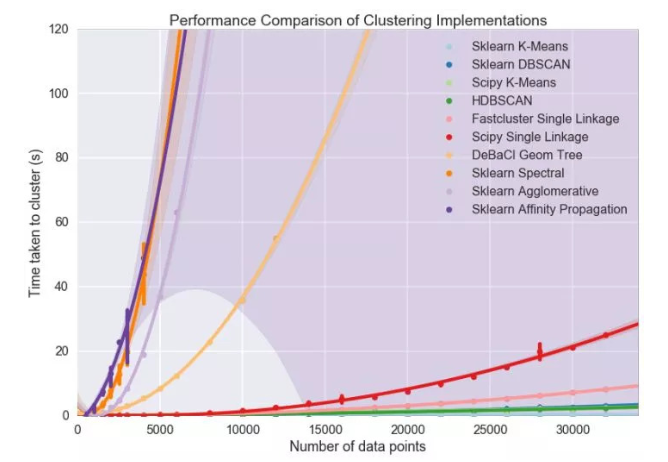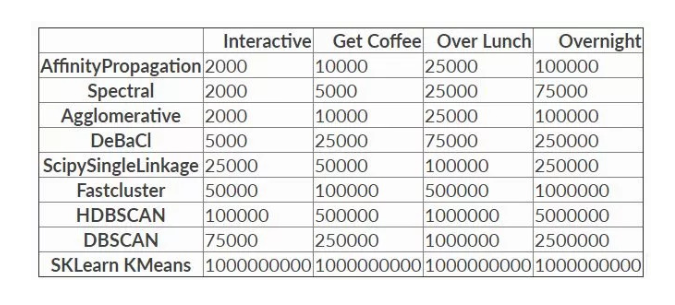.

# 一、scikit-learn中的Kmeans介绍

scikit-learn 是一个基于Python的Machine Learning模块，里面给出了很多Machine
Learning相关的算法实现，其中就包括K-Means算法。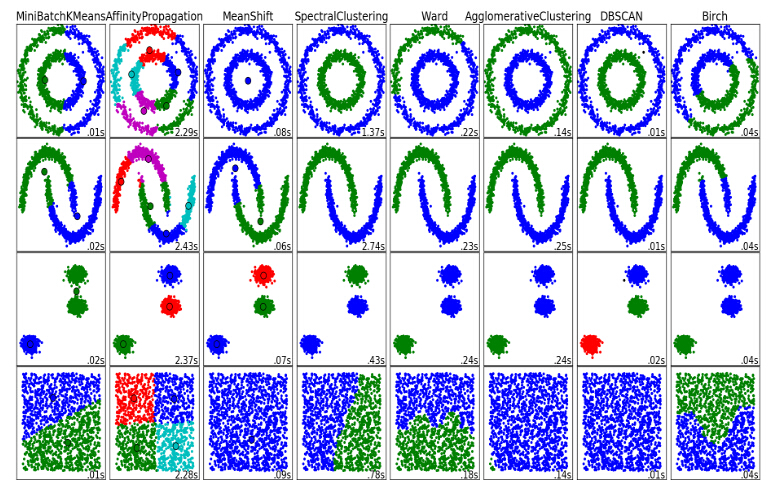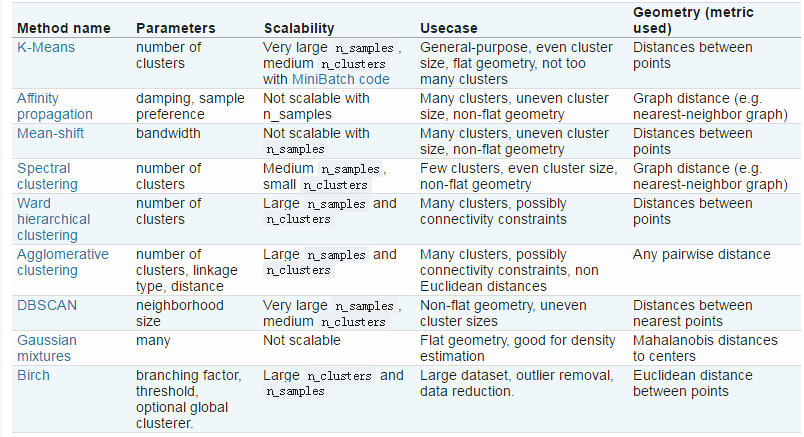优点：



## 1、相关理论

• （1）中心点的选择

k-meams算法的能够保证收敛，但不能保证收敛于全局最优点，当初始中心点选取不好时，只能达到局部最优点，整个聚类的效果也会比较差。可以采用以下方法：k-means中心点

• （2）k值的选取

k-means的误差函数有一个很大缺陷，就是随着簇的个数增加，误差函数趋近于0，最极端的情况是每个记录各为一个单独的簇，此时数据记录的误差为0，但是这样聚类结果并不是我们想要的，可以引入结构风险对模型的复杂度进行惩罚：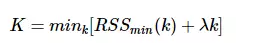λλ是平衡训练误差与簇的个数的参数，但是现在的问题又变成了如何选取λλ了，有研究[参考文献1]指出，在数据集满足高斯分布时，λ=2mλ=2m，其中m是向量的维度。

## 2、主函数KMeans

sklearn.cluster.KMeans(n_clusters=8,
init='k-means++',
n_init=10,
max_iter=300,
tol=0.0001,
precompute_distances='auto',
verbose=0,
random_state=None,
copy_x=True,
n_jobs=1,
algorithm='auto'
)


• n_clusters:簇的个数，即你想聚成几类
• init: 初始簇中心的获取方法
• n_init: 获取初始簇中心的更迭次数，为了弥补初始质心的影响，算法默认会初始10次质心，实现算法，然后返回最好的结果。
• max_iter: 最大迭代次数（因为kmeans算法的实现需要迭代）
• tol: 容忍度，即kmeans运行准则收敛的条件
• precompute_distances：是否需要提前计算距离，这个参数会在空间和时间之间做权衡，如果是True 会把整个距离矩阵都放到内存中，auto 会默认在数据样本大于featurs*samples 的数量大于12e6 的时候False,False 时核心实现的方法是利用Cpython 来实现的
• verbose: 冗长模式（不太懂是啥意思，反正一般不去改默认值）
• random_state: 随机生成簇中心的状态条件。
• copy_x: 对是否修改数据的一个标记，如果True，即复制了就不会修改数据。bool 在scikit-learn 很多接口中都会有这个参数的，就是是否对输入数据继续copy 操作，以便不修改用户的输入数据。这个要理解Python 的内存机制才会比较清楚。
• n_jobs: 并行设置
• algorithm: kmeans的实现算法，有：‘auto’, ‘full’, ‘elkan’, 其中 'full’表示用EM方式实现

## 3、简单案例一

import numpy as np
from sklearn.cluster import KMeans
data = np.random.rand(100, 3) #生成一个随机数据，样本大小为100, 特征数为3

#假如我要构造一个聚类数为3的聚类器
estimator = KMeans(n_clusters=3)#构造聚类器
estimator.fit(data)#聚类
label_pred = estimator.labels_ #获取聚类标签
centroids = estimator.cluster_centers_ #获取聚类中心
inertia = estimator.inertia_ # 获取聚类准则的总和


estimator初始化Kmeans聚类；estimator.fit聚类内容拟合；
estimator.label_聚类标签，这是一种方式，还有一种是predict；estimator.cluster_centers_聚类中心均值向量矩阵
estimator.inertia_代表聚类中心均值向量的总和

## 4、案例二

from sklearn.cluster import KMeans

num_clusters = 3
km_cluster = KMeans(n_clusters=num_clusters, max_iter=300, n_init=40, \
init='k-means++',n_jobs=-1)

#返回各自文本的所被分配到的类索引
result = km_cluster.fit_predict(tfidf_matrix)

print "Predicting result: ", result


km_cluster是KMeans初始化，其中用init的初始值选择算法用’k-means++’；
km_cluster.fit_predict相当于两个动作的合并：km_cluster.fit（data）+km_cluster.predict（data），可以一次性得到聚类预测之后的标签，免去了中间过程。

• n_clusters: 指定K的值
• max_iter: 对于单次初始值计算的最大迭代次数
• n_init: 重新选择初始值的次数
• init: 制定初始值选择的算法
• n_jobs: 进程个数，为-1的时候是指默认跑满CPU
• 注意，这个对于单个初始值的计算始终只会使用单进程计算，
• 并行计算只是针对与不同初始值的计算。比如n_init=10，n_jobs=40,
• 服务器上面有20个CPU可以开40个进程，最终只会开10个进程

km_cluster.labels_
km_cluster.predict(data)


## 5、案例四——Kmeans的后续分析

Kmeans算法之后的一些分析，参考来源：用Python实现文档聚类

from sklearn.cluster import KMeans

num_clusters = 5

km = KMeans(n_clusters=num_clusters)

%time km.fit(tfidf_matrix)

clusters = km.labels_.tolist()


• （1）模型保存与载入
from sklearn.externals import joblib

# 注释语句用来存储你的模型
joblib.dump(km,  'doc_cluster.pkl')
clusters = km.labels_.tolist()

• （2）聚类类别统计
frame = pd.DataFrame(films, index = [clusters] , columns = ['rank', 'title', 'cluster', 'genre'])
frame['cluster'].value_counts()

• （3）质心均值向量计算组内平方和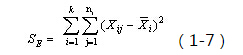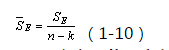km.inertia_


.

**公众号“素质云笔记”定期更新博客内容：**
![这里写图片描述](https://img-blog.csdn.net/20180226155348545?watermark/2/text/aHR0cDovL2Jsb2cuY3Nkbi5uZXQvc2luYXRfMjY5MTczODM=/font/5a6L5L2T/fontsize/400/fill/I0JBQkFCMA==/dissolve/70)

# 二、大数据量下的Mini-Batch-KMeans算法

MiniBatchKmeans 继承自Kmeans 因为MiniBathcKmeans 本质上还利用了Kmeans 的思想.从构造方法和文档大致能看到这些参数的含义，了解了这些参数会对使用的时候有很大的帮助。batch_size 是每次选取的用于计算的数据的样本量，默认为100.

Mini Batch K-Means算法是K-Means算法的变种，采用小批量的数据子集减小计算时间，同时仍试图优化目标函数，这里所谓的小批量是指每次训练算法时所随机抽取的数据子集，采用这些随机产生的子集进行训练算法，大大减小了计算时间，与其他算法相比，减少了k-均值的收敛时间，小批量k-均值产生的结果，一般只略差于标准算法。

1：从数据集中随机抽取一些数据形成小批量，把他们分配给最近的质心
2：更新质心

Mini Batch K-Means比K-Means有更快的 收敛速度，但同时也降低了聚类的效果，但是在实际项目中却表现得不明显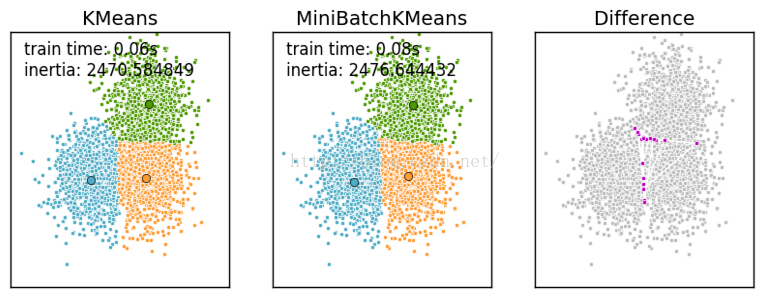### 主函数 :

MiniBatchKMeans(n_clusters=8, init=’k-means++’, max_iter=100, batch_size=100, verbose=0, compute_labels=True, random_state=None,
tol=0.0, max_no_improvement=10, init_size=None, n_init=3, reassignment_ratio=0.01)


• random_state: 随机生成簇中心的状态条件,譬如设置random_state = 9

• tol: 容忍度，即kmeans运行准则收敛的条件

• max_no_improvement：即连续多少个Mini Batch没有改善聚类效果的话，就停止算法，
和reassignment_ratio， max_iter一样是为了控制算法运行时间的。默认是10.一般用默认值就足够了。

• batch_size：即用来跑Mini Batch
KMeans算法的采样集的大小，默认是100.如果发现数据集的类别较多或者噪音点较多，需要增加这个值以达到较好的聚类效果。

• reassignment_ratio:
某个类别质心被重新赋值的最大次数比例，这个和max_iter一样是为了控制算法运行时间的。这个比例是占样本总数的比例，
乘以样本总数就得到了每个类别质心可以重新赋值的次数。如果取值较高的话算法收敛时间可能会增加，尤其是那些暂时拥有样本数较少的质心。
默认是0.01。如果数据量不是超大的话，比如1w以下，建议使用默认值。 如果数据量超过1w，类别又比较多，可能需要适当减少这个比例值。
具体要根据训练集来决定。

import time

import numpy as np
import matplotlib.pyplot as plt

from sklearn.cluster import MiniBatchKMeans, KMeans
from sklearn.metrics.pairwise import pairwise_distances_argmin
from sklearn.datasets.samples_generator import make_blobs

# 获取数据
np.random.seed(0)

batch_size = 45
centers = [[1, 1], [-1, -1], [1, -1]]
n_clusters = len(centers)
X, labels_true = make_blobs(n_samples=3000, centers=centers, cluster_std=0.7)

# kmeans
# Compute clustering with Means

k_means = KMeans(init='k-means++', n_clusters=3, n_init=10)
t0 = time.time()
k_means.fit(X)
t_batch = time.time() - t0

# MiniBatchKMeans

mbk = MiniBatchKMeans(init='k-means++', n_clusters=3, batch_size=batch_size,
n_init=10, max_no_improvement=10, verbose=0)
t0 = time.time()
mbk.fit(X)
t_mini_batch = time.time() - t0



.

# 三、sklearn中的cluster进行kmeans聚类

import numpy as np
from sklearn import cluster
data = np.random.rand(100, 3) #生成一个随机数据，样本大小为100, 特征数为3
k = 3 # 假如我要聚类为3个clusters
[centroid, label, inertia] = cluster.k_means(data, k)


# 四、分类变量聚类方法的K-modes与K-prototype

K-prototype与K-modes

K-modes是K-means用在非数值集合上的一种方法，将原本K-means使用的欧式距离替换成字符间的汉明距离。

K-prototype是K-means与K-modes的一种集合形式，适用于数值类型与字符类型集合的数据。

1. 度量具有混合属性的方法是，数值属性采用K-means方法得到P1，分类属性采用K-modes方法P2，那么D=P1+a*P2，a是权重。如果觉得分类属性重要，则增加a，否则减少a，a=0时即只有数值属性
2. 更新一个簇的中心的方法，方法是结合K-means与K-modes的更新。

code实现可参考：nicodv/kmodes

**公众号“素质云笔记”定期更新博客内容：**
![这里写图片描述](https://img-blog.csdn.net/20180226155348545?watermark/2/text/aHR0cDovL2Jsb2cuY3Nkbi5uZXQvc2luYXRfMjY5MTczODM=/font/5a6L5L2T/fontsize/400/fill/I0JBQkFCMA==/dissolve/70)

.

## 延伸一：数据如何做标准化

data_zs = 1.0*(data - data.mean())/data.std() #数据标准化


.

## 延伸二：Kmeans可视化案例

from sklearn.cluster import KMeans
from sklearn.externals import joblib
import numpy
import time
import matplotlib.pyplot as plt

if __name__ == '__main__':
## step 1: 加载数据
print "step 1: load data..."
dataSet = []
fileIn = open('./data.txt')
for line in fileIn.readlines():
lineArr = line.strip().split(' ')
dataSet.append([float(lineArr), float(lineArr)])

#设定不同k值以运算
for k in range(2,10):
clf = KMeans(n_clusters=k) #设定k  ！！！！！！！！！！这里就是调用KMeans算法
s = clf.fit(dataSet) #加载数据集合
numSamples = len(dataSet)
centroids = clf.labels_
print centroids,type(centroids) #显示中心点
print clf.inertia_  #显示聚类效果
mark = ['or', 'ob', 'og', 'ok', '^r', '+r', 'sr', 'dr', '<r', 'pr']
#画出所有样例点 属于同一分类的绘制同样的颜色
for i in xrange(numSamples):
#markIndex = int(clusterAssment[i, 0])
plt.plot(dataSet[i], dataSet[i], mark[clf.labels_[i]]) #mark[markIndex])
mark = ['Dr', 'Db', 'Dg', 'Dk', '^b', '+b', 'sb', 'db', '<b', 'pb']
# 画出质点，用特殊图型
centroids =  clf.cluster_centers_
for i in range(k):
plt.plot(centroids[i], centroids[i], mark[i], markersize = 12)
#print centroids[i, 0], centroids[i, 1]
plt.show()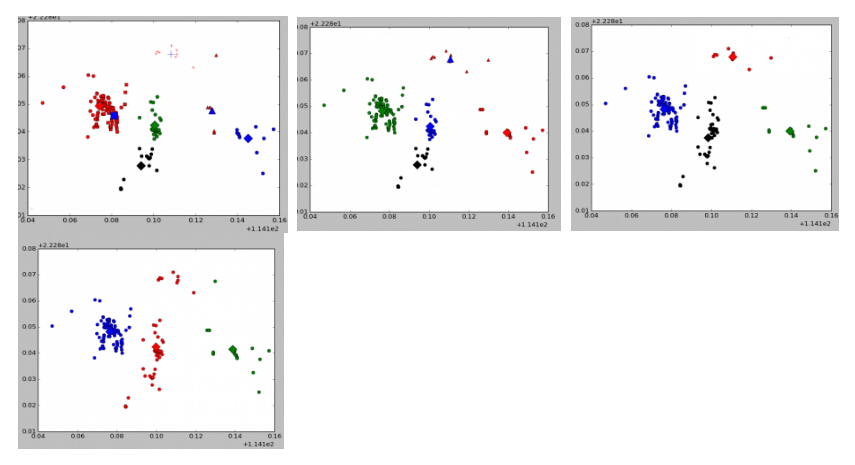## 延伸三：模型保存

from sklearn.externals import joblib
joblib.dump(km_cluster, "/..../train_model.m")
kmeans_SSE.labels_


## 延伸四：HDBSCAN与Kmeans的聚类的一些纪要